Next: Polarization Vectors Up: QED Processes Previous: Coulomb Scattering of Positrons

# Photons

Maxwell's equations of classical electrodynamics in vacuum are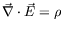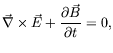(7.88)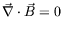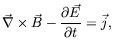where we are using Heaviside-Lorentz (rationalized Gaussian) units.

Defining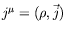and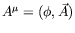, the four-vector potential is related to the electric and magnetic fields by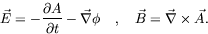(7.89)

Further, we can show that in terms of the antisymmetric field strength tensor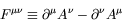(7.90)

that Maxwell's equations now take the compact form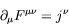(7.91)

and current conservation,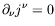, follows as a natural compatibility condition.

These equations are equivalent to the following covariant equation for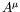.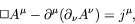(7.92)

which is general andis in the presence of charge and currents.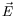and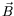are unchanged by the gauge transformation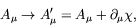(7.93)

where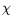is any scaler function of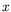. We use this freedom to pickso that we can write Maxwell's equations in the form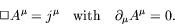(7.94)

The requirement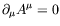is known as the Lorentz condition. The process of choosing a particular condition onso as to define it uniquely is called choosing a gauge''. Potentials satisfyingare said to be in the Lorentz gauge''. However, even after choosing a gauge, there is still some residual freedom in the choice of the potential. We can still make another gauge transformation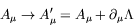(7.95)

where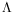is any function that satisfies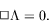(7.96)

This last equation ensures that the Lorentz condition is still satisfied.

SubsectionsNext: Polarization Vectors Up: QED Processes Previous: Coulomb Scattering of Positrons
Douglas M. Gingrich (gingrich@ ualberta.ca)
2004-03-18